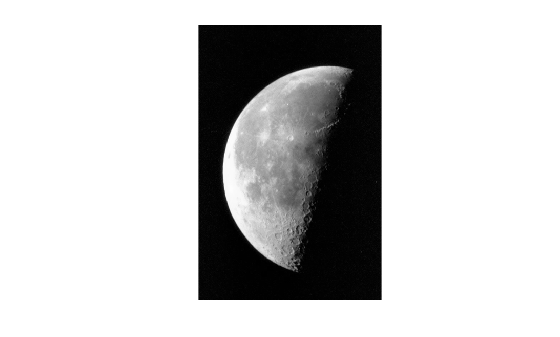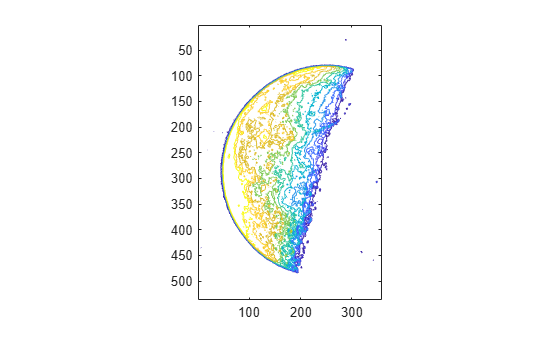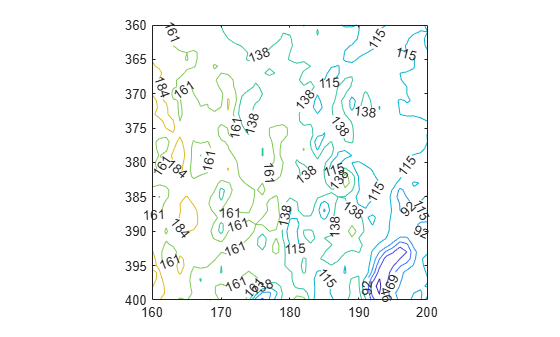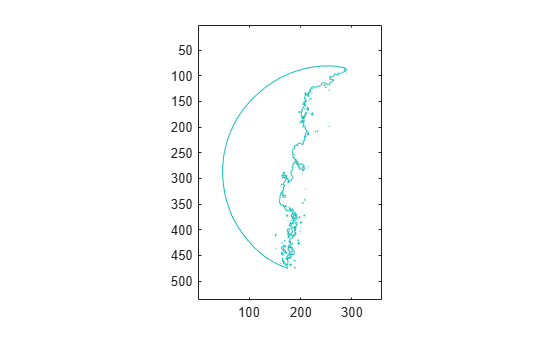# Create Contour Plot of Grayscale Image

This example shows how to create a contour plot of an image. A contour is a path in an image along which the image intensity values are equal to a constant. You can create a contour plot of the data in a grayscale image using the `imcontour` function. This function is similar to the `contour` function in MATLAB®, but it automatically sets up the axes so their orientation and aspect ratio match the image.

Read and display a grayscale image.

```I = imread("moon.tif"); imshow(I)```Create a contour plot of the image with ten contour levels using `imcontour`.

`[C,h] = imcontour(I,10);`Display the levels that the `imcontour` function selected.

`h.LevelList`
```ans = 1×10 23 46 69 92 115 138 161 184 207 230 ```

To label the levels of the contours, use the `clabel` function. Zoom in to see the contour details.

```clabel(C,h) xlim([160 200]) ylim([360 400])```Display a single contour at level 128.

`imcontour(I,[128 128]);`